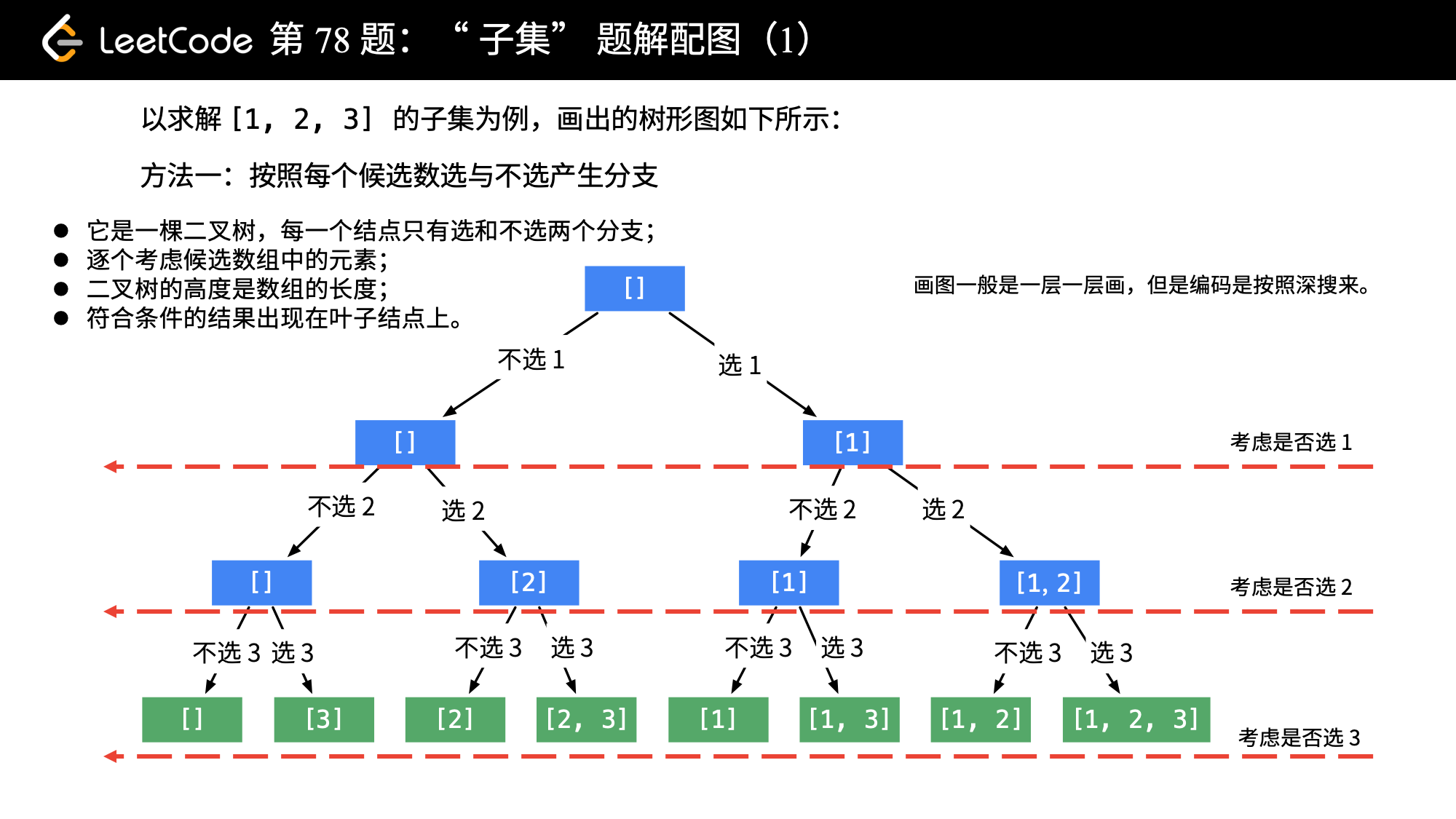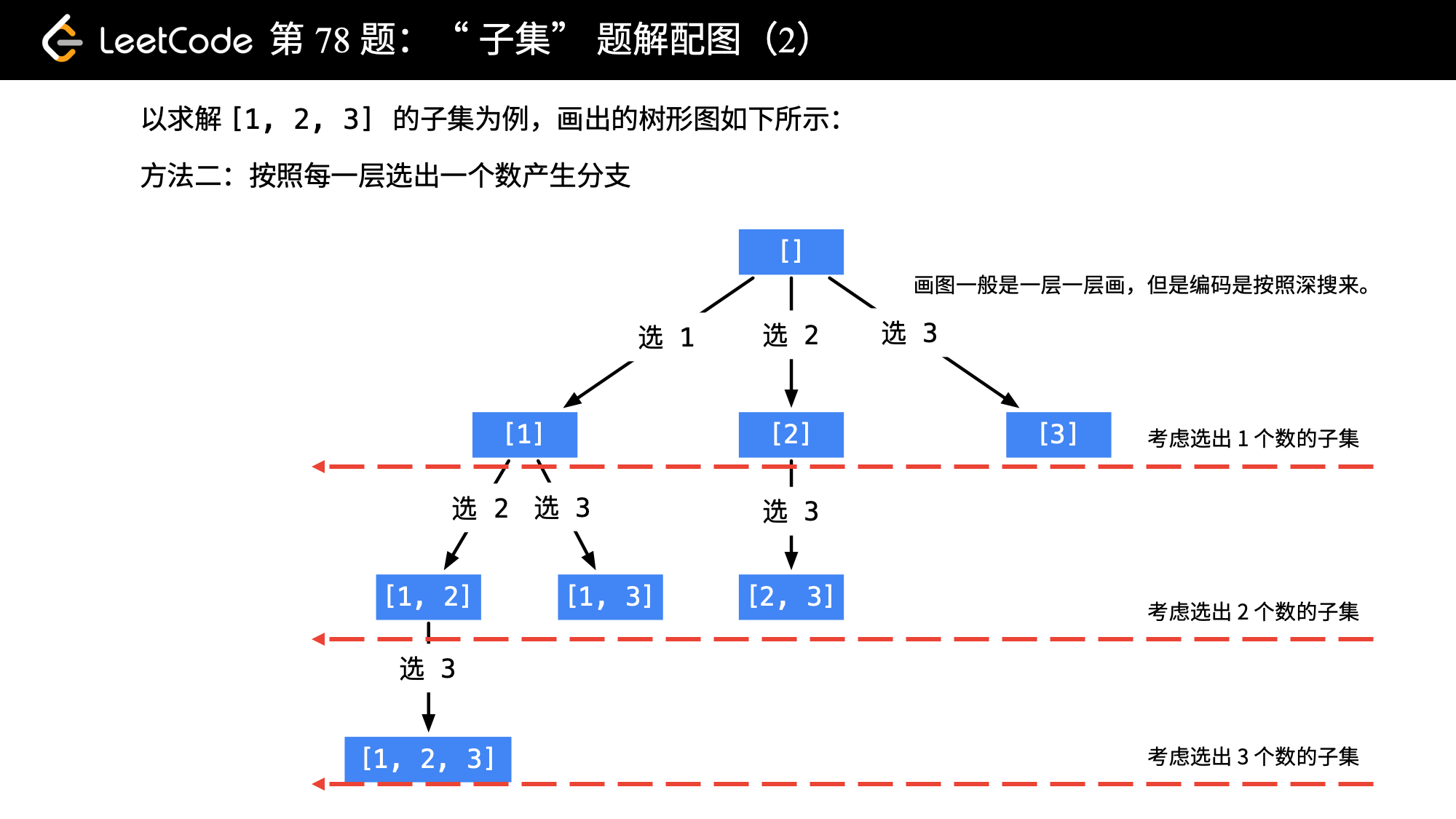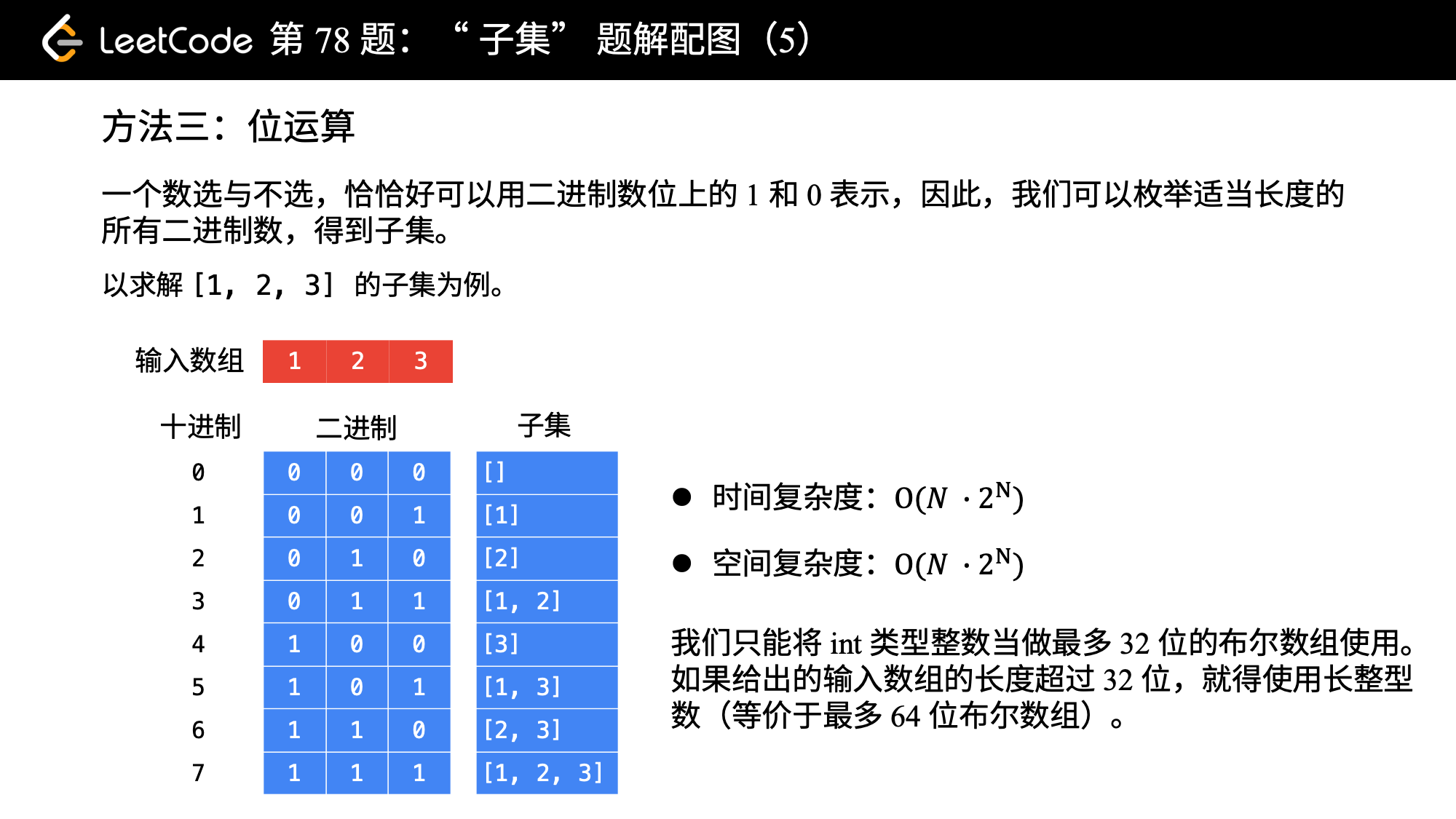# 「力扣」第 78 题：子集（中等）

## 「力扣」第 78 题：子集（中等）

输入: nums = [1,2,3]

[
,
,
,
[1,2,3],
[1,3],
[2,3],
[1,2],
[]
]

• 画出树形图：按照“一个数可以选，也可以不选”的思路，画出如下树形图；• 结果出现在哪里？所有符合条件的结果出现在叶子结点中。
• 使用深度优先遍历需要的状态变量：1、当前考虑的是第几个数 index；2、从根结点到叶子结点的路径 path ，不难分析出它是一个栈。

Java 代码：

import java.util.ArrayDeque;
import java.util.ArrayList;
import java.util.Deque;
import java.util.List;

public class Solution {

public List<List<Integer>> subsets(int[] nums) {
int len = nums.length;

List<List<Integer>> res = new ArrayList<>();
if (len == 0){
return res;
}

Deque<Integer> path = new ArrayDeque<>();
dfs(nums, len, 0, path, res);
return res;
}

private void dfs(int[] nums, int len, int index, Deque<Integer> path, List<List<Integer>> res) {
if (index == len){
return;
}

dfs(nums, len, index + 1, path, res);
path.removeLast();

dfs(nums, len, index + 1, path, res);
}
}

[[], , , [2, 3], , [1, 3], [1, 2], [1, 2, 3]]

• 时间复杂度：$O(N \times 2^N)$，这里 $N$ 为数组的长度，叶子结点一共有 $2^N$ 个，树的高度为 $N$。
• 空间复杂度：$O(N \times 2^N)$，理由同时间复杂度。保存子集需要长度为 $2^N$ 的列表，每一个子集的元素最多长度为 $N$。

• 画出树形图：按照“按照每一层选出一个数产生分支”的思路，可以画出如下树形图；• 结果出现在哪里？所有的结点都是符合条件的结果。
• 使用深度优先遍历需要的状态变量：1、从候选数组的哪一个下标开始搜索 start；2、从根结点到叶子结点的路径 path ，这个变量我们多次遇到了。

Java 代码：

import java.util.ArrayDeque;
import java.util.ArrayList;
import java.util.Deque;
import java.util.List;

public class Solution {

public List<List<Integer>> subsets(int[] nums) {
List<List<Integer>> res = new ArrayList<>();
int len = nums.length;
if (len == 0) {
return res;
}

Deque<Integer> path = new ArrayDeque<>();
dfs(nums, 0, len, path, res);
return res;
}

private void dfs(int[] nums, int begin, int len, Deque<Integer> path, List<List<Integer>> res) {
// 在遍历的过程中，收集符合条件的结果
for (int i = begin; i < len; i++) {
dfs(nums, i + 1, len, path, res);
path.removeLast();
}
}
}

• 时间复杂度：$O(2^N)$，整棵树的结点个数一共是 $2^N$ 个。
• 空间复杂度：$O(N \times 2^N)$，保存子集需要长度为 $2^N$ 的列表，每一个子集的元素最多长度为 $N$。

• 每一个候选数选与不选，这恰恰好是计算机世界里二进制数能够表示的含义，1 表示选择，0 表示不选；
• 因此，我们可以枚举数组长度的二进制数的所有可能十进制值，按照每一个数位的值枚举所有的可能性（这句话没有说得很准确，大家领会意思即可）。Java 代码：

import java.util.ArrayList;
import java.util.List;

public class Solution {

public List<List<Integer>> subsets(int[] nums) {
int size = nums.length;
int n = 1 << size;
List<List<Integer>> res = new ArrayList<>();

for (int i = 0; i < n; i++) {
List<Integer> cur = new ArrayList<>();
for (int j = 0; j < size; j++) {
if (((i >> j) & 1) == 1) {
}
}
}
return res;
}
}

• 时间复杂度：$O(N \times 2^N)$，这里 $N$ 为数组的长度，叶子结点一共有 $2^N$ 个子集，遍历每一个子集所代表的二进制数有 $N$ 位。
• 空间复杂度：$O(N \times 2^N)$，保存子集需要长度为 $2^N$ 的列表，每一个子集的元素最多长度为 $N$。

上一篇「力扣」第 90 题：子集 II
「力扣」第 90 题：子集 II 链接 题解链接 给定一个可能包含重复元素的整数数组 nums，返回该数组所有可能的子集（幂集）。 说明：解集不能包含重复的子集。 示例： 输入：nums = [1,2,3] 输出： [ , [
2018-02-16「力扣」第 77 题：组合（中等）
「力扣」第 77 题：组合（中等） 链接 题解链接 给定两个整数 n 和 k，返回 1 ... n 中所有可能的 k 个数的组合。 示例： 输入: n = 4, k = 2 输出: [ [2, 4], [3, 4], [2,
2018-02-14
目录